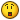# Script help...

## Recommended Posts

Ok i have a script that relogs in as differnet accounts, but when i get over 9 u have a problem because of two digits.

how would i make it so if \$AccountNumber > 9 then add a 0 in front of the number. or just have the numbers go like this 001,002,003,004,005,006,007,008,009,010,011....

##### Share on other sites
• Moderators

Something like this?

`If \$AccountNumber > 9 Then \$AccountNumber = 0 & \$AccountNumber`

Or for the second one like this:

```If \$AccountNumber <= 9 Then \$AccountNumber = 0 & 0 & \$AccountNumber
If \$AccountNumber > 9 Then \$AccountNumber = 0 & \$AccountNumber```
##### Share on other sites

Something like this?

`If \$AccountNumber > 9 Then \$AccountNumber = 0 & \$AccountNumber`

Or for the second one like this:

```If \$AccountNumber <= 9 Then \$AccountNumber = 0 & 0 & \$AccountNumber
If \$AccountNumber > 9 Then \$AccountNumber = 0 & \$AccountNumber```

So it will produce this?

```\$AccountNumber = 50
If \$AccountNumber > 9 Then \$AccountNumber = 0 & \$AccountNumber```

Account #: 050

and

```\$AccountNumber = 4
\$AccountNumber <= 9 Then \$AccountNumber = 0 & 0 & \$AccountNumber```

Account #: 004

?

##### Share on other sites

yea but what ill happen when i do

\$AccountNumber = \$AccountNumber + 1

Do the zeros interfear? or can i just remove them, like strip all the zeros before adding them.

Edited by blizzedout
##### Share on other sites
• Moderators

So it will produce this?

```\$AccountNumber = 50
If \$AccountNumber > 9 Then \$AccountNumber = 0 & \$AccountNumber```

Account #: 050

and

```\$AccountNumber = 4
\$AccountNumber <= 9 Then \$AccountNumber = 0 & 0 & \$AccountNumber```

Account #: 004

?

Yes it will...if you expect to go over 100 account you will have to allow for that:

```\$AccountNumber = 100
If \$AccountNumber <= 9 Then \$AccountNumber = 0 & 0 & \$AccountNumber
If \$AccountNumber > 9 And \$AccountNumber < 100 Then \$AccountNumber = 0 & \$AccountNumber```
##### Share on other sites

Yes it will...if you expect to go over 100 account you will have to allow for that:

```\$AccountNumber = 100
If \$AccountNumber <= 9 Then \$AccountNumber = 0 & 0 & \$AccountNumber
If \$AccountNumber > 9 And \$AccountNumber < 100 Then \$AccountNumber = 0 & \$AccountNumber```
Na i dont need it to go over 100 but thanksjust wana know if the zeros will interfear when doing:

\$AccountNumber = \$AccountNumber + 1

##### Share on other sites
• Moderators

Na i dont need it to go over 100 but thanksjust wana know if the zeros will interfear when doing:

\$AccountNumber = \$AccountNumber + 1

Well...try this, it seems to work fine for me:

```\$AccountNumber = 20
If \$AccountNumber <= 9 Then \$AccountNumber = 0 & 0 & \$AccountNumber
If \$AccountNumber > 9 And \$AccountNumber < 100 Then \$AccountNumber = 0 & \$AccountNumber
InputBox("", "", \$AccountNumber)
\$AccountNumber = \$AccountNumber + 1
If \$AccountNumber <= 9 Then \$AccountNumber = 0 & 0 & \$AccountNumber
If \$AccountNumber > 9 And \$AccountNumber < 100 Then \$AccountNumber = 0 & \$AccountNumber
InputBox("", "", \$AccountNumber)```
##### Share on other sites

Works good thank man, tested it by doin this.

```\$AccountNumber = 0
While 1
\$AccountNumber = \$AccountNumber + 1
If \$AccountNumber <= 9 Then \$AccountNumber = 0 & 0 & \$AccountNumber
If \$AccountNumber > 9 Then \$AccountNumber = 0 & \$AccountNumber
MsgBox(4096, "Test", "Account #: " & \$AccountNumber, 10)
Wend```

Turns out when you add 1 to 001 it will produce 2 not 002## Create an account

Register a new account

• ### Recently Browsing   0 members

×

• Wiki

• Back

• #### Beta

• Git
• FAQ
• Our Picks
×
• Create New...